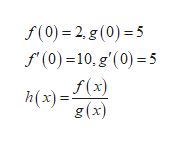Consider the functions f(x) and g(x), for which f(0) = 2, g(0) = 5, f'(0) = 10, and g'(0) = 5.Find h'(0) for the function h(x) = f(x) / g(x).h'(0) = ?

Question

Consider the functions f(x) and g(x), for which f(0) = 2, g(0) = 5, f'(0) = 10, and g'(0) = 5.

Find h'(0) for the function h(x) = f(x) / g(x).

h'(0) = ?

Step 1help_outlineImage Transcriptionclosef(0) 2,g (0) 5 f (0) 10,g'(0) =5 f(x) i(х)- g (x) fullscreen

Want to see the full answer?

See Solution

Want to see this answer and more?

Our solutions are written by experts, many with advanced degrees, and available 24/7

See Solution
Tagged in

Calculus